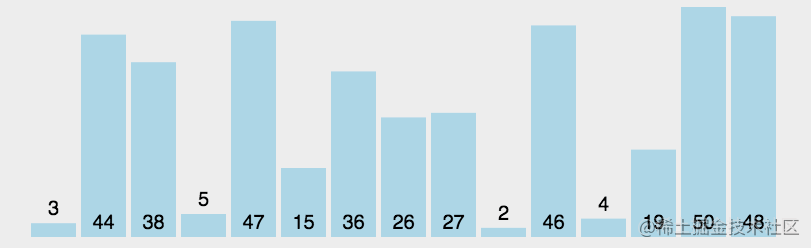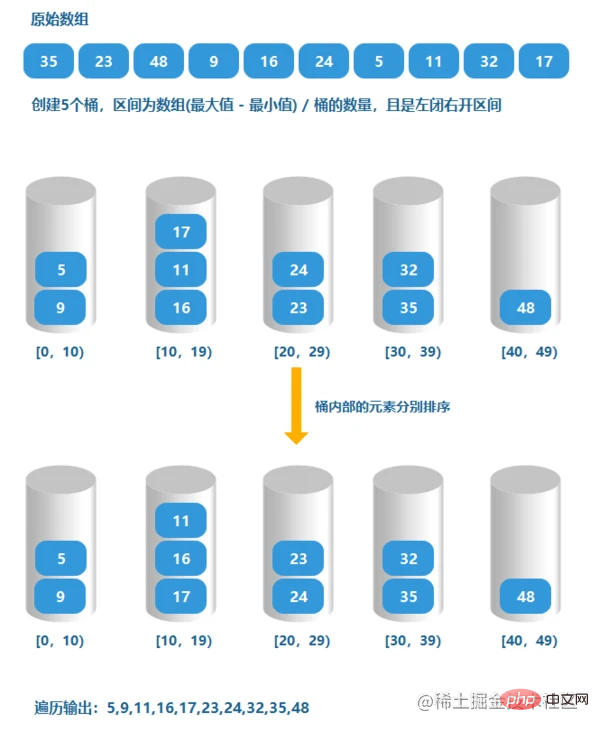# JavaScript实现十大排序算法（图文详解）【相关推荐：javascript视频教程web前端

## 冒泡排序

### 排序的效果图### 解法

```function bubbleSort(arr){
const len = arr.length;
for(let i = 0; i < len - 1; i++){
for(let j = 0; j < len - i - 1; j++){
if(arr[j] > arr[j+1]){
const tmp = arr[j+1];
arr[j+1] = arr[j];
arr[j] = tmp;
}
}
}

return arr;
}```

## 快速排序

### 效果图### 解法

```function quickSort(arr){

sort(arr, 0, arr.length - 1);
return arr;

function sort(arr, low, high){
if(low >= high){
return;
}

let i = low;
let j = high;
const x = arr[i]; // 取出比较值x，当前位置i空出，等待填入
while(i < j){
// 从数组尾部，找出比x小的数字
while(arr[j] >= x && i < j){
j--;
}
// 将空出的位置，填入当前值， 下标j位置空出
// ps：比较值已经缓存在变量x中
if(i < j){
arr[i] = arr[j]
i++;
}

// 从数组头部，找出比x大的数字
while(arr[i] <= x && i < j){
i++;
}
// 将数字填入下标j中，下标i位置突出
if(i < j){
arr[j] = arr[i]
j--;
}
// 一直循环到左右指针i、j相遇，
// 相遇时，i==j, 所以下标i位置是空出的
}

arr[i] = x; // 将空出的位置，填入缓存的数字x，一轮排序完成

// 分别对剩下的两个区间进行递归排序
sort(arr, low, i - 1);
sort(arr, i+1, high);
}
}```

## 希尔排序

### 效果图### 解法

```function shellSort(arr){
// 分组规则 gap/2 递减
for(let gap = Math.floor(arr.length/2); gap > 0; gap = Math.floor(gap/2)){
for(let i = gap; i < arr.length; i++){
let j = i;
// 分组内数字，执行插入排序，
// 当下标大的数字，小于 下标小的数字，进行交互
// 这里注意，分组内的数字，并不是一次性比较完，需要i逐步递增，囊括下个分组内数字
while(j - gap >= 0 && arr[j] < arr[j - gap]){
swap(j, j-gap);
j = j - gap;
}
}
}

return arr;

function swap(a, b){
const tmp = arr[a];
arr[a] = arr[b];
arr[b] = tmp;
}
}```

```function shellSort(arr){

for(let gap = Math.floor(arr.length/2); gap > 0; gap = Math.floor(gap/2)){
for(let i = gap; i < arr.length; i++){
let j = i;
const x = arr[j]; // 缓存数字，空出位置

while(j - gap >= 0 && x < arr[j-gap]){
arr[j] = arr[j - gap]; // 将符合条件的数字，填入空出的位置
j = j - gap;
}
arr[j] = x; // 最后，将缓存的数字，填入空出的位置
}
}

return arr;
}```

## 选择排序

### 排序的效果图### 解法

```function selectionSort(arr){
const len = arr.length;

for(let i = 0; i < len-1; i++){
let minIndex = i;
for(let j = i+1; j < len; j++){
if(arr[j] < arr[minIndex]){
minIndex = j; // 保存最小数的下标
}
}

const tmp = arr[i];
arr[i] = arr[minIndex];
arr[minIndex] = tmp;
}

return arr;
}```

## 归并排序

### 效果图### 小数组合并的过程### 解法

```function mergeSort(arr){

return sort(arr, 0, arr.length - 1); // 注意右区间是arr.length - 1

// sort方法，进行递归
function sort(arr, left, right){

// 当left !== right时，证明还没分拆到最小元素
if(left < right){
// 取中间值，分拆为两个小的数组
const mid = Math.floor((left+right) / 2);
const leftArr = sort(arr, left, mid);
const rightArr = sort(arr, mid+1, right);
// 递归合并
return merge(leftArr, rightArr)
}

// left == right, 已经是最小元素，直接返回即可
return left >= 0 ? [arr[left]] : [];
}

// 合并两个有序数组
function merge(leftArr, rightArr){
let left = 0;
let right = 0;
const tmp = [];

// 使用双指针，对两个数组进行扫描
while(left < leftArr.length && right < rightArr.length){
if(leftArr[left] <= rightArr[right]){
tmp.push(leftArr[left++]);
}else{
tmp.push(rightArr[right++]);
}
}

// 合并剩下的内容
if(left < leftArr.length){
while(left < leftArr.length){
tmp.push(leftArr[left++]);
}
}

if(right < rightArr.length){
while(right < rightArr.length){
tmp.push(rightArr[right++]);
}
}

return tmp;
}

}```

## 插入排序

### 排序的效果图### 解法

```function insertionSort(arr){
const len = arr.length;

// 注意，i 从 1 开始
for(let i = 1; i < len; i++){
let preIndex = i - 1;
let current = arr[i];

// 位置i之前，是已排好序的数字，while的作用是找到一个坑位，给当前数字current插入
while(preIndex >= 0 && arr[preIndex] > current){
arr[preIndex+1] = arr[preIndex]; // 对大于current的值，往后移一位，给current的插入腾出位置
preIndex--;
}
arr[preIndex+1] = current;
}

return arr;
}```

## 堆排序

### 概要• 通过构建大顶堆

• 将堆顶的最大数拿出，与堆底的叶子节点进行交换

• 接着，树剪掉最大数的叶子

• 再对堆进行调整，重新变成大顶堆

• 返回步骤2，以此循环，直至取出所有数

### 效果图### 解法

```function heapSort(arr){

// 初次构建大顶堆
for(let i = Math.floor(arr.length/2) - 1; i >= 0; i--){
// 开始的第一个节点是 树的最后一个非叶子节点
// 从构建子树开始，逐步调整
buildHeap(arr, i, arr.length);
}

// 逐个抽出堆顶最大值
for(let j = arr.length -1 ; j > 0; j--){
swap(arr, 0, j); // 抽出堆顶（下标0）的值，与最后的叶子节点进行交换
// 重新构建大顶堆
// 由于上一步的堆顶最大值已经交换到数组的末尾，所以，它的位置固定下来
// 剩下要比较的数组，长度是j,所以这里的值length == j
buildHeap(arr, 0, j);
}

return arr;

// 构建大顶堆
function buildHeap(arr, i, length){
let tmp = arr[i];

for(let k = 2*i+1; k < length; k = 2*k+1){
// 先判断左右叶子节点，哪个比较大
if(k+1 < length && arr[k+1] > arr[k]){
k++;
}
// 将最大的叶子节点，与当前的值进行比较
if(arr[k] > tmp){
// k节点大于i节点的值，需要交换
arr[i] = arr[k]; // 将k节点的值与i节点的值交换
i = k; // 注意：交换后，当前值tmp的下标是k，所以需要更新
}else{
// 如果tmp大于左右子节点，则它们的子树也不用判断，都是小于当前值
break;
}

}

// i是交换后的下标，更新为tmp
arr[i] = tmp;
}

// 交换值
function swap(arr, i, j){
const tmp = arr[i];
arr[i] = arr[j];
arr[j] = tmp;
}
}```

## 计数排序

### 效果图### 解法

```function countingSort(arr){
let maxValue = Number.MIN_VALUE;
let minValue = Number.MAX_VALUE;
let offset = 0; // 位移，用于处理负数
const result = [];

// 取出数组的最大值, 最小值
arr.forEach(num => {
maxValue = num > maxValue ? num : maxValue;
minValue = num > minValue ? minValue : num;
});

if(minValue < 0){
offset = -minValue;
}

const bucket = new Array(maxValue+offset+1).fill(0); // 初始化连续的格子

// 将数组中的每个数字，根据值放入对应的下标中，
// `bucket[num] == n`格子的意义：存在n个数字，值为num
arr.forEach(num => {
bucket[num+offset]++;
});

// 读取格子中的数
bucket.forEach((store, index) => {
while(store--){
result.push(index - offset);
}
});

return result;

}```

## 桶排序

### 效果图### 解法

```function bucketSort(arr, bucketSize = 10){
// bucketSize 每个桶可以存放的数字区间(0, 9]

if(arr.length <= 1){
return arr;
}

let maxValue = arr;
let minValue = arr;
let result = [];

// 取出数组的最大值, 最小值
arr.forEach(num => {
maxValue = num > maxValue ? num : maxValue;
minValue = num > minValue ? minValue : num;
});

// 初始化桶的数量
const bucketCount = Math.floor((maxValue - minValue)/bucketSize) + 1; // 桶的数量
// 初始化桶的容器
// 注意这里的js语法，不能直接fill([])，因为生成的二维下标数组，是同一个地址
const buckets = new Array(bucketCount).fill(0).map(() => []);

// 将数字按照映射的规则，放入桶中
arr.forEach(num => {
const bucketIndex = Math.floor((num - minValue)/bucketSize);
buckets[bucketIndex].push(num);
});

// 遍历每个桶内存储的数字
buckets.forEach(store => {
// 桶内只有1个数字或者空桶，或者都是重复数字，则直接合并到结果中
if(store.length <= 1 || bucketSize == 1){
result = result.concat(store);
return;
}

// 递归，将桶内的数字，再进行一次划分到不同的桶中
const subSize = Math.floor(bucketSize/2); // 减少桶内的数字区间，但必须是最少为1
const tmp = bucketSort(store, subSize <= 1 ? 1: subSize);
result = result.concat(tmp);
});

return result;
}```

## 基数排序

### 概述

• MSD 从高位开始进行排序

• LSD 从低位开始进行排序

### 效果图### 解法

```function radixSort(arr){
let maxNum = arr;

// 求出最大的数字，用于确定最大进制位
arr.forEach(num => {
if(num > maxNum){
maxNum = num;
}
});

// 获取最大数字有几位
let maxDigitNum = 0;
while(maxNum > 0){
maxNum = Math.floor(maxNum / 10);
maxDigitNum++;
}

// 对每个进制位上的数进行排序
for(let i = 0; i < maxDigitNum; i++){
let buckets = new Array(10).fill(0).map(() => []); // 初始化10个桶
for(let k = 0; k < arr.length; k++){
const bucketIndex = getDigitNum(arr[k], i); // 获取当前进制位上的数字
buckets[bucketIndex].push(arr[k]); // 排序的数字放入对应桶中
}
// 所有数字放入桶中后，现从0-9的顺序将桶中的数字取出
const res = [];
buckets.forEach(store => {
store.forEach(num => {
res.push(num); // 注意这里，先存入桶中的数字，先取出，这样才能保持局部有序
})
});

arr = res;
}

return arr;

/**
求出数字每个进制位上的数字，只支持正整数
@param num 整数
@param digit 位数，从0开始
*/
function getDigitNum(num, digit){
return Math.floor(num / Math.pow(10, digit) % 10)
}
}```

## 算法复杂度【相关推荐：javascript视频教程web前端• 相关标签：javascript
• 相关文章

相关视频